﻿ Forces
﻿

## Attractive Forces

m1,m2: mass of two objects; r: distance between two objects; G: gravitational constant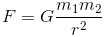## Gravitational Force

M: Earth mass, m: mass of object, R: Earth radius, h: object’s height## Gravitational Acceleration

At a height h above the normal surface of the earth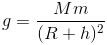Near the sea level## Force Of Gravity

Force of gravity on earth, a special case of Gravitational force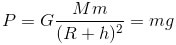## Newton's First Law

F: forces acting on the object , v: velocity of the object, a: acceleration of the object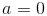## Newton's Second Law

m: object’s mass(kg)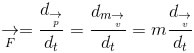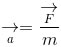## Newton's Third Law## Linear Elasticity-Hooke's Law

K: spring constant (n/m), x: displacement(m)A Mass suspended by a spring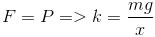## Force Of friction

µ: coefficient of friction, N: normal force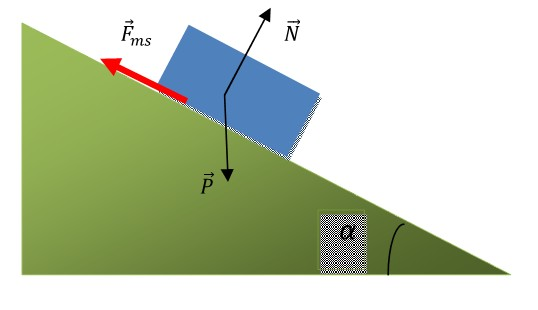Fms = µN
= µPcosα
= µmgcosα